## 2012年1月13日星期五

### Evaluating Measurement Uncertainty (Part 1)

Hong Kong Accreditation Service (HKAS) arranged "Analytical Quality Training Programme" from 9 to 13 Jan 2012. LGC experts were invited to provide this training. LGC is the UK’s designated National Measurement Institute for chemical and biochemical analysis , the National Reference Laboratory for a range of key areas, and is also the host organisation for the UK’s Government Chemist function.

The third training course named "Evaluating Measurement Uncertainty" from 12 to 13 Jan 2012. The training content was summarized for sharing.

Measurement Uncertainty (Part 1 on 12 Jan 2012)

In the beginning, Ms. Vicki Barwick introduced What and Why to evaluate measurement uncertainty.She quoted the ISO definition of Measurement Uncertainty as "A parameter, associated with the result of a measurement, that characterises the dispersion of the values that could reasonably be attributed to the measurand"Ms. Barwick explained the different between Error and Uncertainty.

- ERROR is a difference (measured value - true value).

- UNCERTAINTY is a range.

- ERROR needs to know a true value, but UNCERTAINTY needs not be known.

Then she showed the comparison of different lab results based on precision and uncertainty.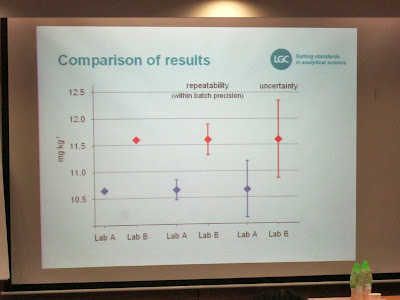After the introduction, Dr. Stephen Ellison performed a review session called "Statistics refresher". It is because measurement uncertainty estimation relies in part on statistical principles.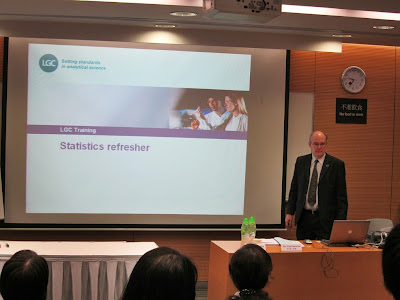He introduced different distributions (i.e. Normal, Rectangular and Triangular) which were commonly used in calculating measurement uncertainty. And then mean, sample standard deviation (s), relative standard deviation (rsd), standard deviation of the mean (sdm) and confidence intervals were briefed.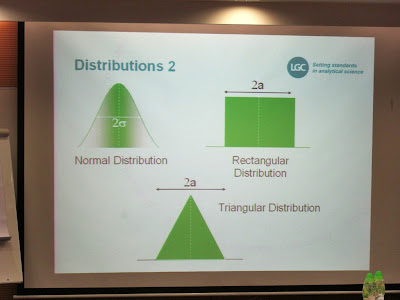Next session named "ISO measurement uncertainty principles" was presented by Ms. Vicki Barwick.The background to the ISO Guide was briefed. The earliest ISO Guide had published since 1993. It is now very widely accepted because of globalization trades.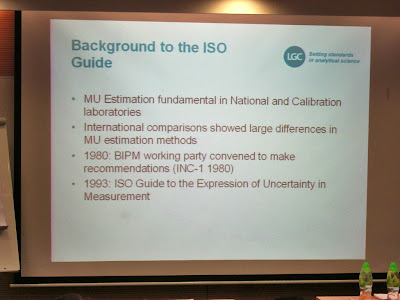She said ISO recommended that uncertainties arose from several contributions (as standard deviation) and two ways of evaluating uncertainty components. They were statistical (Type A) and otherwise (Type B) uncertainty. The following assumptions were used in this ISO approach. It should be expressed as "expanded uncertainty" for additional confidence.Dr. Stephen Ellison introduced some rules for uncertainty calculation included converting data to standard uncertainties and combining uncertainties. Firstly, he briefed standard deviation of the mean (s/√n) and the calculation of confidence interval (x + (t * s)/√n). (Details please see the training summary on 9 Jan 2012)The most common Type B standard uncertainty was rectangular distribution and calculation was showed below (a/3).In combining uncertainties, sensitivity coefficient was discussed. It is the gradient of the line relating y and xi (variation).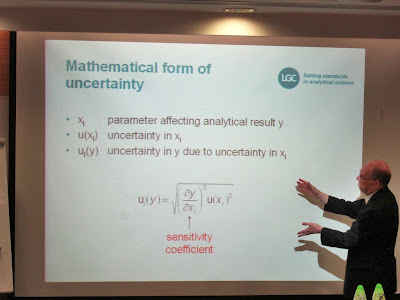The diagram illustrated how to calculate the uncertainty in a measured volume due to change in temperature. It is a very good example to explain the sensitivity coefficient in graphic method.He said the combination of uncertainties had four types. They were Addition, Subtraction, Product and Quotient. Combination of uncertainties for Addition or Subtraction were to square root the sum of square for all standard uncertainties which had already considered the sensitivity coefficient. For Product or Quotient, the uncertainties were expressed as relative standard deviations (See diagram below).Finally, the expanded uncertainty was combined uncertainty times coverage factor (k). k was set to 2 for most practical purposes.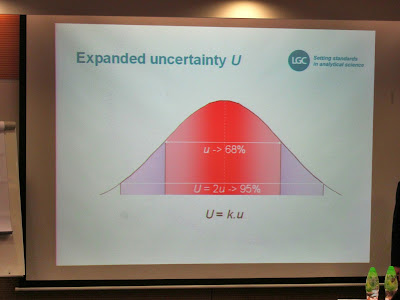The next session was "Quantifying Uncertainty Components" and Ms. Vicki Barwick explained it in five dimensions. They were Random Variation, Systematic Variation, Calculation, Published Information and Experience.She discussed the random variation. In nested design experiment, ANOVA was the normal way of analysing the data.Then she discussed the systematic variation using regression analysis. The experimental sensitivity coefficient (gradient) was calculated.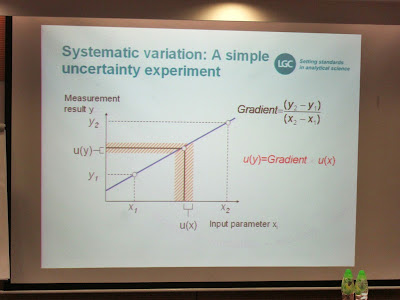Numerical calculation was introduced. The uncertainty from individual factor (e.g. Volume) could be identified by the difference between concentration due to uncertainty in the volume and original concentration calculated without considered such uncertainty. Using spreadsheets to calculate all factors' uncertainty and then calculate the combined uncertainty. This calculation had already included sensitivity coefficient.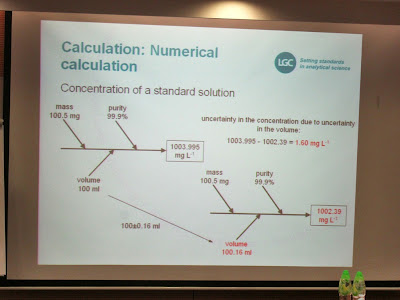After that Dr. Stephen Ellison taught us to use spreadsheet to evaluating uncertainty.At the end, Ms. Vicki Barwick discussed how to handle precision data. There were two method to estimate precision:

1. By combining variability from input parameters (e.g. Replicate weighting of a check weight)

2. By directly observing output variability (e.g. Reproducibility data from a collaborative trial)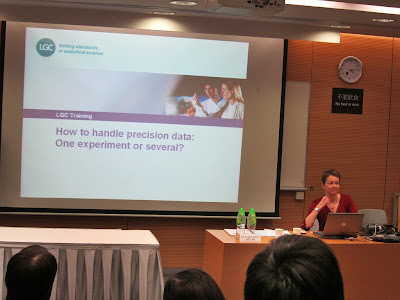Then she introduced two approaches to dealing with precision. It was either to evaluate the individual precision terms or to obtain a single overall precision estimate for the method as a whole.Reference:

HKAS - www.hkas.gov.hk

ISO/IEC 17025: General requirements for the competence of testing and calibration laboratories

UKAS M3003: The Expression of uncertainty and confidence in measurement - www.ukas.com

EA-4/16 EA Guidelines on the expression of uncertainty in quantitative testing - www.european-accreditation.org

ILAC G17:2002 Introducing the concept of uncertainty of measurement in testing in association with the application of the standard ISO/IEC 17025 - www.ilac.org